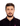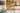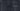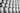Navigate back to the homepage

# .css-1mo6ayw{display:block;opacity:0;-webkit-transform-origin:center top;-ms-transform-origin:center top;transform-origin:center top;-webkit-transform-style:preserve-3d;-ms-transform-style:preserve-3d;transform-style:preserve-3d;-webkit-transform:translateY(100%) rotateX(-80deg);-ms-transform:translateY(100%) rotateX(-80deg);transform:translateY(100%) rotateX(-80deg);-webkit-transition:opacity 0s cubic-bezier(0.215,0.61,0.355,1),-webkit-transform 0s cubic-bezier(0.215,0.61,0.355,1);-webkit-transition:opacity 0s cubic-bezier(0.215,0.61,0.355,1),transform 0s cubic-bezier(0.215,0.61,0.355,1);transition:opacity 0s cubic-bezier(0.215,0.61,0.355,1),transform 0s cubic-bezier(0.215,0.61,0.355,1);}html.has-scroll-init .css-1mo6ayw{opacity:1;-webkit-transition-duration:0.8s;transition-duration:0.8s;-webkit-transform:none;-ms-transform:none;transform:none;}Useful utilities functions to use in your next JavaScript project

### a collection of useful functions that you can use to create or expand your utilities library!Ahmed Abdulrahman
Jan 2nd, 2020 • ☕️ 1 min read
javascriptPhoto by Barn Images on Unsplash

### Check if all values in an array are equal

You can check it using `every` method on the array:

`1const areEqual = arr => {2  return arr.length > 0 && arr.every(item => item === arr);3}4// areEqual([11, 32, 21, 54]) === false 🚫5// areEqual(['blue', 'blue', 'blue']) === true ✅`

### Convert an array of strings value to numbers

JS provides different ways to convert a string value into a number, but the best one is to use `Number` object in a non-constructor context (without the new keyword). It also takes care of the decimals as well:

`1const toNumbers = arr => arr.map(Number);2// toNumbers(["2", "3", "4"]) returns [2, 3, 4]`

### Create an array of cumulative sum

To get the summation of a sequence of numbers in an array, we can use currying to access the previous value:

`1const accumulate = arr => {2  return arr.map((sum => value => sum += value)(0));3}45/*6accumulate([1, 2, 3, 4]) === [1, 3, 6, 10]7// 1             = 18// 1 + 2         = 39// 1 + 2 + 3     = 610// 1 + 2 + 3 + 4 = 1011*/`

### Create an array of numbers in the given range

To create an array that contains all numbers between `starting index` and `ending index`, including `starting index` and `ending index` themselves:

`1const range = (min, max) => {2  return Array(max - min + 1).fill(0).map((_, i) => max - min + i)3};4// range(5, 10) === [5, 6, 7, 8, 9, 10]`

### Empty an array

JS provides various ways of clearing existing array:

`1const empty = arr => arr.length = 0;23// Or using splice but since the .splice() function will return an array4// with all the removed items, it will actually return a copy of5// the original array6const empty = arr => arr.splice(0, arr.length)78// Or9arr = [];`

### Find the maximum or minimum item of an array

`1const max = arr => Math.max(...arr);2const min = arr => Math.min(...arr);`

### Check if an object is an array

`1const isArray = obj => Array.isArray(obj);`

### Get a random item from an array

`1const randomItem = arr => {2  return arr[Math.floor(Math.random() * arr.length)]3};`

### Get the average of an array

`1const average = arr => {2  return arr.reduce((a, b) => a + b, 0) / arr.length;3}`

### Get the sum of array of numbers

`1const sum = arr => arr.reduce((a, b) => a + b, 0);`

### Get the unique values of an array

`1const unique = arr => [...new Set(arr)];23// Or4const unique = arr => {5  return arr.filter((el, i, array) => array.indexOf(el) === i);6}`

### Merge two arrays

We can achieve this in different ways:

`1// 1 - To just merge the arrays (without removing duplicates)2const merge = (a, b) => a.concat(b);3// OR4const merge = (a, b) => [...a, ...b];56// 2 -  To merge the arrays and removing duplicates7const merge = [...new Set(a.concat(b))];8// OR9const merge = [...new Set([...a, ...b])];`

I hope this blog would have piqued your interest in knowing more about these helper methods. Go ahead, try them out and share with others.

Cheers !

### Other things I've writtenLet's talk about essential tools and apps for development

Oct 16th, 2018 · 4 min read## ADD vs COPY in Docker

In this post, we'll learn what are ADD and COPY commands in docker, How they differ, and why COPY is more secure than ADD.

Mar 17th, 2020 · 1 min read